# Basic functions - math word problems

#### Number of problems found: 2267

• Playing cards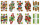From 32 playing cards containing 8 red cards, we choose 4 cards. What is the probability that just 2 will be red?
• SickSick Marcel already taken six tablets, which was a quarter of the total number of pills in the pack. How many pills were in the pack?
• How much 2How much money would you need to deposit today at 5% annual interest compounded monthly to have \$2000 in the account after 9 years?
• Boys and girlsThere are 20 boys and 10 girls in the class. How many different dance pairs can we make of them?
• Sum and roundingI know two numbers whose sum is 20. When they each round and then added together I get the 30. What are this numbers?
• The confectionery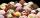The confectionery sold 5 kinds of ice cream. In how many ways can I buy 3 kinds if order of ice creams does not matter?
• Cyclist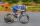A cyclist passes 88 km in 4 hours. How many kilometers did he pass in 8 hours?
• Butter fat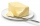A quarter of kg butter contains 82% fat. How many grams of fat are in four cubes of butter?
• BracketsPlace one pair of brackets in expression 20-16 + 10 - 4 - 2 so that the result will be 0.
• Salary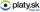Calculate the equivalent annual salary to an hourly wage of \$ 19. Assume a 42-hour workweek.
• Gold coin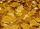Gold coin contains 964 promile pure gold, which is 7.16 g. What is the weight of coin in grams?
• Powers 3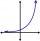2 to the power of n divided by 4 to the power of -3 equal 4. What is the vaule of n?
• Divisible by nineHow many three-digit natural numbers in total are divisible without a remainder by the number 9?
• Cube edgesIf the edge length of the cube increases by 50%, how does the volume of this cube increase?
• Secret number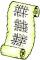Determine the secret number n, which reversed decrease by 16.4 if the number increase by 16.4.
• Pump680 liters of water were pumped in 8 minutes. How many liters was spent in 56 minutes?
• Sixty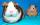Sixty percent of one-fifteen of the total is equal to thirty. What are two percent of the total?
• GirlsThe children's competition was attended by 63 girls, which is 30% of all children's participants. How many children attended this competition?On a straight stretch of road is marked 12 percent drop. What angle makes the direction of the road with the horizontal plane?Gardeners planted 16% more currant bushes than originally planned. In total, they planted x = 435 shrubs. What was the original plan?